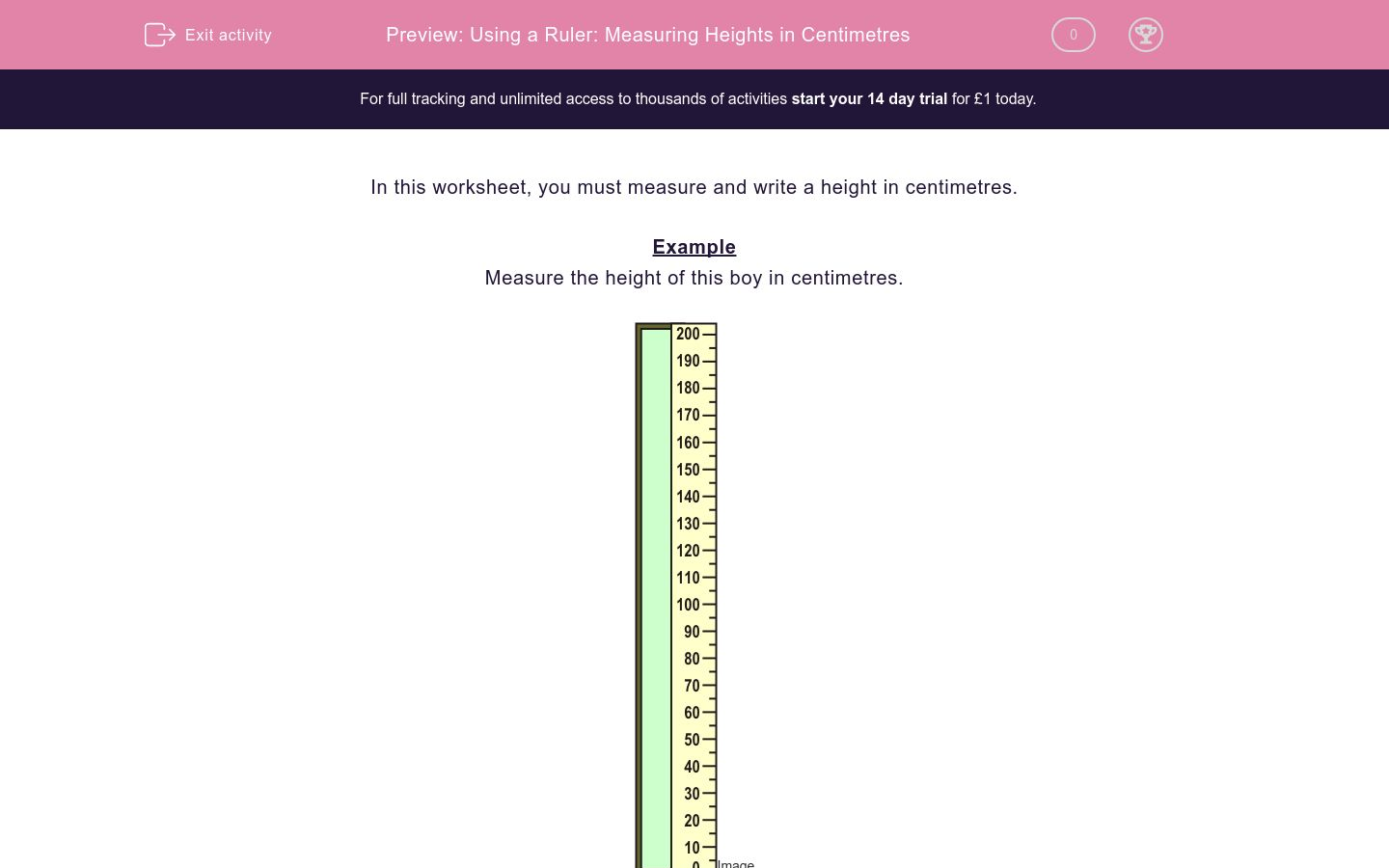# Using a Ruler: Measuring Heights in Centimetres

In this worksheet, students measure a height in centimetres by reading a scale on a ruler.Key stage:  KS 2

Curriculum topic:   Maths and Numerical Reasoning

Difficulty level:### QUESTION 1 of 10

In this worksheet, you must measure and write a height in centimetres.

Example

Measure the height of this boy in centimetres.The top of the boy's head is level with 100 centimetres on the scale.

Measure the height in centimetres of this boy.

Just write the number.Measure the height in centimetres of this bean stalk.

Just write the number.Measure the height in centimetres of this boy.

Just write the number.Measure the height in centimetres of this ladder.

Just write the number.Measure the height in centimetres of this person.

Just write the number.Measure the height in centimetres of this ladder.

Just write the number.Measure the height in centimetres of this bean stalk.

Just write the number.Measure the height in centimetres of this boy.

Just write the number.Measure the height in centimetres of this boy.

Just write the number.Measure the height of this ladder in centimetres.

Just write the number.• Question 1

Measure the height in centimetres of this boy.

Just write the number.100
• Question 2

Measure the height in centimetres of this bean stalk.

Just write the number.130
• Question 3

Measure the height in centimetres of this boy.

Just write the number.140
• Question 4

Measure the height in centimetres of this ladder.

Just write the number.120
• Question 5

Measure the height in centimetres of this person.

Just write the number.150
• Question 6

Measure the height in centimetres of this ladder.

Just write the number.80
• Question 7

Measure the height in centimetres of this bean stalk.

Just write the number.170
• Question 8

Measure the height in centimetres of this boy.

Just write the number.140
• Question 9

Measure the height in centimetres of this boy.

Just write the number.120
• Question 10

Measure the height of this ladder in centimetres.

Just write the number.140
---- OR ----

Sign up for a £1 trial so you can track and measure your child's progress on this activity.

### What is EdPlace?

We're your National Curriculum aligned online education content provider helping each child succeed in English, maths and science from year 1 to GCSE. With an EdPlace account you’ll be able to track and measure progress, helping each child achieve their best. We build confidence and attainment by personalising each child’s learning at a level that suits them.

Get started